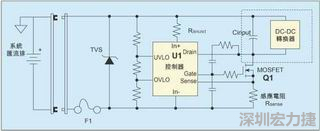简体中文English设为首页加入收藏

0755-83328032

## 高速数位通讯系统的热插拔电路设计元件选择指南PRshunt=(Vinmax-Vregmin)2/Rshunt

Rshunt=(Vinmin-Vrefmax)/2.5mA=35V/2.5mA=14KΩ。

PRshunt=(75V-9V)2/10KΩ=0.44W

IInput max=Pout max/(Vin min×η)
=72W/(36V×0.72)=2.8A

P=Imax2×Rds(on)

P=Ipk2×Rds(on)max=2.82×0.03=0.24W

Ifaultworstcase=Vinmax/(Rds(on)+Rfuse)=75V/(0.030+0.035)=1154A

Pwrshortcircuit=Vinmax×Imax=75V×180A=13,500W公司名称： * 姓名： * 电话： * 邮箱： * 留言内容：